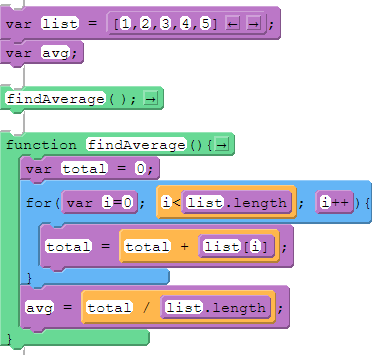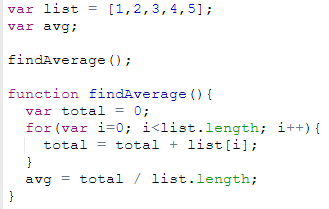# List Reduce Pattern

This pattern is used when you want to reduce the information in a list down to a single number. For example, you might want to find the average of a list of numbers or the smallest number.

Name Code (block) Code (text)
List Reduce - average### How it works

• Create a list of numbers
• Create a blank variable to store the average
• In a function create a local variable to store the total value of all elements in the list
• Use a for loop to access each element in the list and add its value to total
• After the for loop, calculate the average by dividing total by the length of the list
Name Code (block) Code (text)
List Reduce - smallest### How it works

• Create a list of numbers
• Create a blank variable to store the smallest number
• In a function create a local variable to store a temporary value
• Use a for loop to access each element in the list
• If that element is less than the the value stored in temp, set the value of temp to the current element
• After the for loop set the value of smallest to the value stored in temp

Found a bug in the documentation? Let us know at documentation@code.org